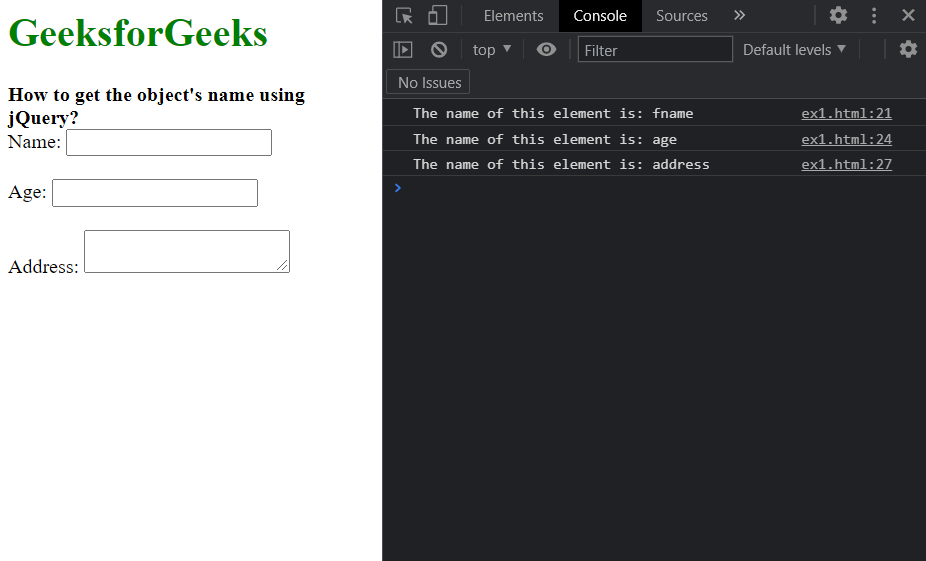Open In App

# How to get the object’s name using jQuery ?

In this article, we will learn how to find the name of an element using jQuery. The name attribute can be applied to multiple elements in HTML and is used to specify a name for any element. The name attribute of any element can be found out using the attr() method. This method is used to find the value of any attribute of the first element in the matched set.

As we need to find the name of an element, we will use this method on the required element and pass “name” as the parameter in this method.

Syntax:

```// Select the required element
let selectedElem = \$("elemToSelect");

// Get the name of the element
// using the attr() method
let elementName = selectedElem.attr('name');

console.log("The name of this element is:",
elementName);```

The example below illustrates the above approach:

Example: In this example, we will create a clone of the object using this method.

## HTML

 ```<``html``>` `<``head``>``    ``<``script` `src``=``        ``"https://code.jquery.com/jquery-3.6.0.min.js"``>``    ````` `<``body``>``    ``<``h1` `style``=``"color: green;"``>``        ``GeeksforGeeks``    ``` `    ``<``b``>``        ``How to get the object's name``        ``using jQuery?``    ````    ` `    ``<``form``>``        ``<``label` `for``=``"name"``>Name:``        ``<``input` `type``=``"text"` `id``=``"name"` `name``=``"fname"` `/>``        ``<``br``><``br``>``        ` `        ``<``label` `for``=``"age"``>Age:``        ``<``input` `type``=``"number"` `id``=``"age"` `name``=``"age"` `/>``        ``<``br``><``br``>``        ` `        ``<``label` `for``=``"address"``>Address:``        ``<``textarea` `id``=``"address"` `name``=``"address"``>``    ``` `    ``<``script``>``    ` `        ``// Select the required element``        ``let selectedElem = \$("#name");` `        ``// Get the name of the element``        ``// using the attr() method``        ``console.log("The name of this element is:",``            ``selectedElem.attr('name'));` `        ``let selectedElem2 = \$("#age");``        ``console.log("The name of this element is:",``            ``selectedElem2.attr('name'));` `        ``let selectedElem3 = \$("#address");``        ``console.log("The name of this element is:",``            ``selectedElem3.attr('name'));``    ````` ``

Output: